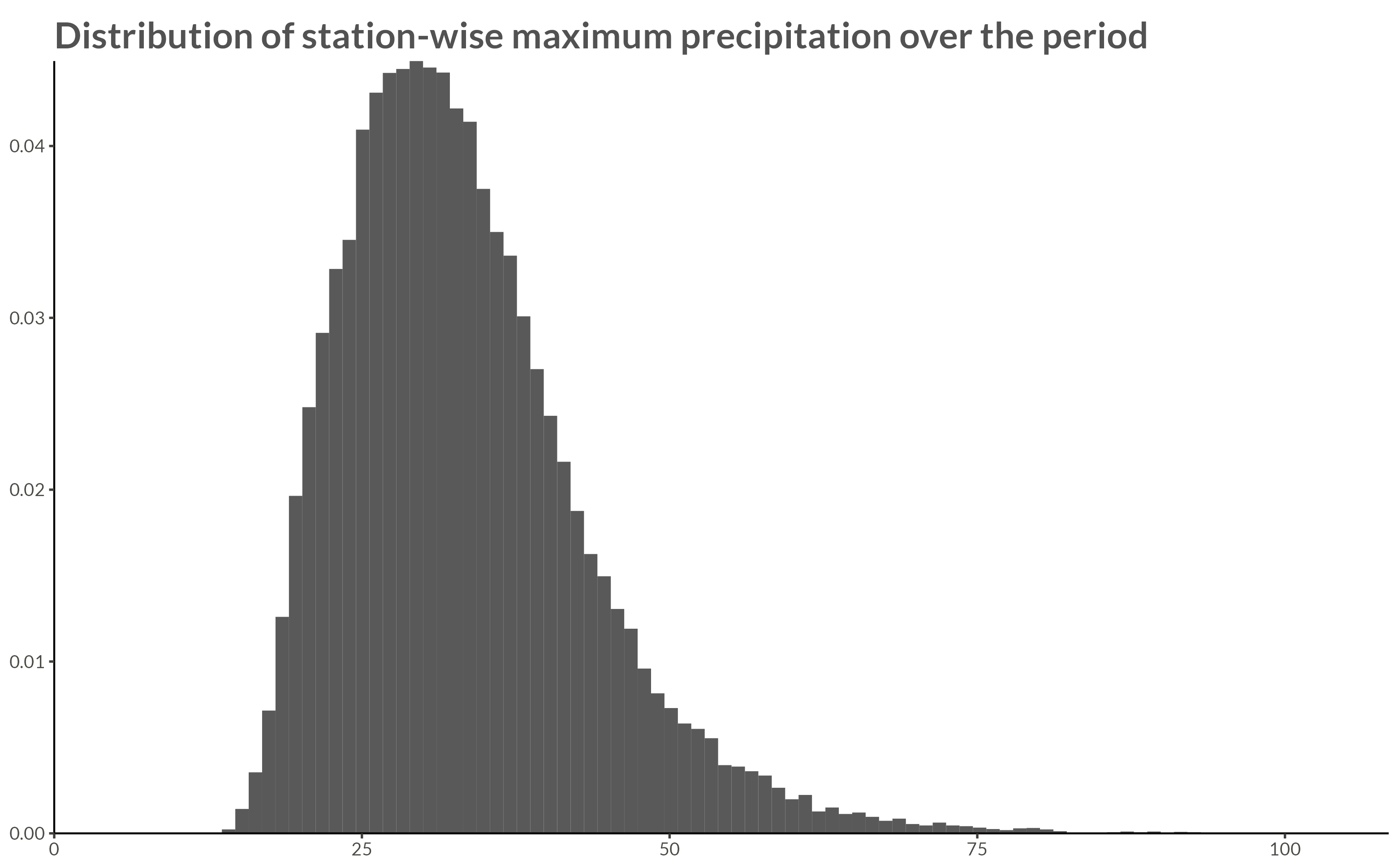# UKCP Data

Code
library(bggjphd)
library(tidyverse)
library(GGally)
library(cowplot)
library(glue)
library(leaflet)
theme_set(theme_bggj())

# Summary

The data used in this research are downloaded from the CEDA Archive:

The raw data contain climate projections for the UK on a 5km grid from 1980 to 2080 for a high emissions scenario, RCP8.5, and contain hourly precipitation rates of $$43.920$$ squares on a $$180 \times 244$$ grid. The projections are calculated for $$1980 - 2000$$, $$2020 - 2040$$, and $$2060 - 2080$$, thus giving $$60 \times 365 \times 24 \times 180 \times 244 \approx 2.3 \cdot 10^{10}$$ data points.

The raw data were processed by calculating the yearly maximum over the hourly precipitation rates for each station, thus reducing the number of data points to $$60 \times 180 \times 244 \approx 2.6 \cdot 10^6$$.

# Summary statistics

## Maximum precipitation

Code
p <- full_data |>
ggplot(aes(max_precip, y = after_stat(density))) +
geom_histogram(bins = 100) +
scale_x_continuous(
limits = c(0, NA),
expand = expansion()
) +
scale_y_continuous(
expand = expansion()
) +
labs(
x = NULL,
y = NULL,
title = "Distribution of station-wise maximum precipitation over the period"
)

ggsave(
plot = p,
filename = "Figures/figure1.png",
width = 8, height = 0.621 * 8, scale = 1.3
)Code
full_data |>
stations_to_sf() |>
points_to_grid() |>
spatial_plot(max_precip)